# Fit of only a part of total area in TGraph2D

Hello,

I would like to make a fit of the TGraph2D that you see in Figure 1. When I use a 2D polynomial function I get the result presented in Figure 2. I think that the reason is that part of the area in the graph has no data (the violet area in the plot).

I would like to make a fit only of the area with data. The problem is that it is not a square region that I could define setting the range. Is it possible to define a range using a function instead of fixed values?

In general, does somebody know how to force the fit procedure to ignore the area with no data?

Estela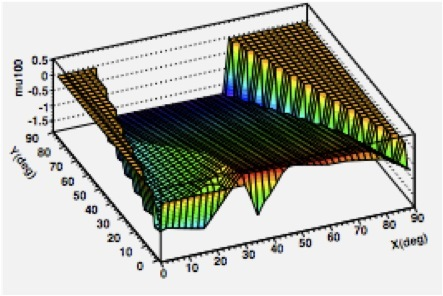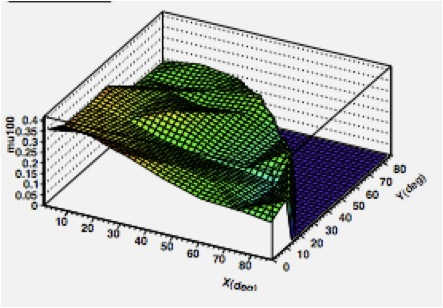Hello,
the region with no data is always excluded when fitting a TGraph2D.
What you observe is probably that the function is extrapolated in the region with no data and it is drawn in figure 2.
If it makes sense, you can probably change this by
defining better your function, i.e. to be a polynomial in the data region and a null function outside.

Best Regards

Lorenzo

Hi,

I tried to fit just a polynomial function, of the type:

```TF2 *f2 = new TF2("f2", "+*x+*x*x+*y+*y*y+*x*y",0.,90,0.,90.); ```

I tried applying it directly, and also defining it as a user function in the way:

[code]Double_t fitf(Double_t *x, Double_t *par)
{
//x contains the x value
//x contains the y value
//par
// +*x+xx+*y+yy+xy
Double_t fitval(0);

if ( sqrt(x*x+x*x)<90. )
{
fitval=par+par*x+par*x*x+par*x+par*x*x+par*x*x;
} else {fitval=0;}

return fitval;
}
[/code]

putting in this second case in the body of my program:

``TF2 *f2 = new TF2("f2","fitf",0.,90,0.,90.);``

In both cases I get the result of the figure 3. Figure 3a is the distribution that I want to fit. Figure 3b shows the result of the fit function together with the points to be fitted (white dots).

Any suggestion to get a better fit will be welcomed.

Estela.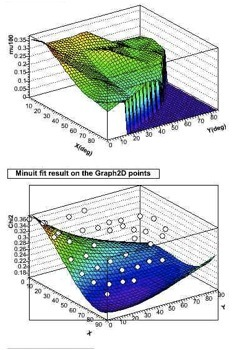Hi,

can you post your macro and a root file containing your TGraph2D object ?
Thank you

Lorenzo

Hi,

sure. I attach three files:

• graph.root: its the TGraph2D that I posted in previous figure 3b.
• grapherr.root: the same, but plotted as TGraph2DErrors.
• macro.C: the macro to fit and make the plot of the data.

I send “grapherr.root” just in case that it would work better considering the errors of the data points. I have tried it myself and the result was not better.

The macro reads by default “graph.root”. If you’d like to use the errors, just comment the first two lines and uncomment the following two.

Thanks a lot for your attention,
Estela.
macro.C (1.94 KB)
grapherr.root (11.7 KB)
graph.root (11 KB)

Hi,

see the attached macro. You must create the fit function correctly, not using a formula but as a C function

Lorenzo
macro.C (2.39 KB)

Thanks a lot Lorenzo.

Just a couple of questions related with its graphical representation:

-When I plot the points and the fit together, a thin black line appears as a frame of the graph (see figure below). How can I remove it?

-I’d like to show the scale of the color palette at the side of the graph. How can I do this? I know that when plotting a histogram I can access the palette through TPaletteAxis, but I don’t know how to access this class from a TGraph2D.

Thanks again,
Estela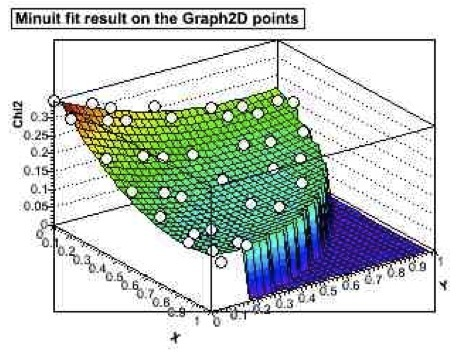I cannot get this picture with the posted macro.C (the last one) can you send me the macro.C producing this exact picture ?

Hi,

attached is the macro. (I think in the old macro the sentence g->Draw(“same p0”) was just commented)

Thanks,
Estela
macro.C (2.39 KB)

with your macro I get this: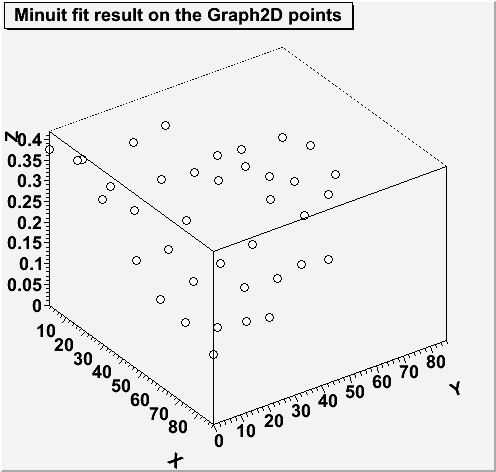That’s strange, because I get the figure below

I am using root 5.20.00 in a Linux Fedora system, maybe that can make the difference

[code]

• ``````                                    *
``````
• ``````   W E L C O M E  to  R O O T       *
``````
• ``````                                    *
``````
• Version 5.20/00 24 June 2008 *
• ``````                                    *
``````
• ``````     http://root.cern.ch            *
``````
• ``````                                    *
``````

ROOT 5.20/00 (trunk@24524, Jun 26 2008, 14:53:00 on linux)

CINT/ROOT C/C++ Interpreter version 5.16.29, Jan 08, 2008
Type ? for help. Commands must be C++ statements.
Enclose multiple statements between { }.[/code]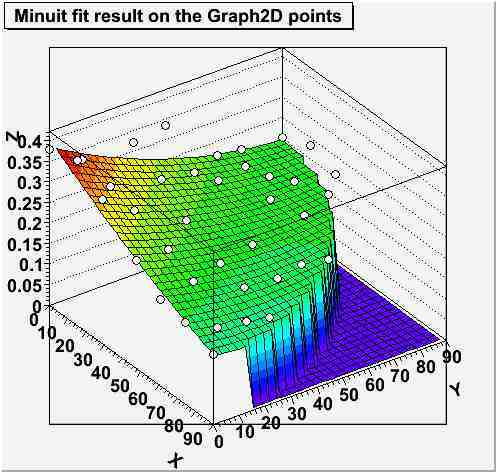Here is a simplified " macro.C" doing what you asked for.
macro.C (984 Bytes)

Thanks a lot, that’s what I needed.

Last question: is there a way to access the TPaletteAxis from the TF2 directly, so that I can define it’s size, position and others? I’ve been able to do it with the intermediate step of creating a histogram, as I show below.

[code] TH2D h = (TH2D)f2->CreateHistogram();
h->Draw(“surf1 Z”);
h->SetTitle(“Minuit fit result on the Graph2D points”);
h->GetXaxis()->SetTitle(“X”);
h->GetXaxis()->SetTitleOffset(2.0);
h->GetYaxis()->SetTitle(“Y”);
h->GetYaxis()->SetTitleOffset(2.0);
h->GetZaxis()->SetTitle(“Z”);
h->GetZaxis()->SetTitleOffset(1.2);
g->Draw(“same p0”);

c1->Update();
TPaletteAxis plte = (TPaletteAxis)h->GetListOfFunctions()->FindObject(“palette”);
plte->SetX1NDC(0.85);
plte->SetX2NDC(0.87);
plte->SetY1NDC(0.3);
plte->SetY2NDC(0.67);

c1->Modified();
c1->Update();[/code]

It is fine for me to do it as above. I just wondered if a more direct way exists. I tried things like:

``(TPaletteAxis*)f2->GetListOfFunctions()->FindObject("palette");``

but the function GetListOfFunctions doesn’t exist for a TF2 object.

Thanks a lot for all the help proportioned.

Cheers,
Estela.

As you can see here:
root.cern.ch/root/html/TF2.html
GetListOfFunctions is not a member function of TF2. So you should use the 1st solution.

Just to let you know…
Although the method TGraph2D::GetListOfFunctions() exists, getting the palette requires GetHistogram()

Cheers,
Z